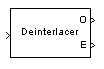# Deinterlacer

Distribute elements of input vector alternately between two output vectors

• Library:
• Communications Toolbox / Sequence Operations

•## Description

The Deinterlacer block accepts an even length column vector input signal. The block alternately places the elements in two output vectors. As a result, each output vector size is half the input vector size. The outputs inherit the sample time from the Sample time parameter of the input block.

## Ports

### Input

expand all

Input signal, specified as an even length column vector.

Data Types: `double` | `single`
Complex Number Support: Yes

### Output

expand all

Odd-numbered elements of the input vector, returned as a vector. If the input is a vector of length N, the output length is N/2.

Even-numbered elements of the input vector, returned as a vector. If the input is a vector of length N, the output length is N/2.

## Block Characteristics

 Data Types `Boolean` | `double` | `enumerated` | `fixed point` | `integer` | `single` Multidimensional Signals `no` Variable-Size Signals `no`

## Version History

Introduced before R2006a